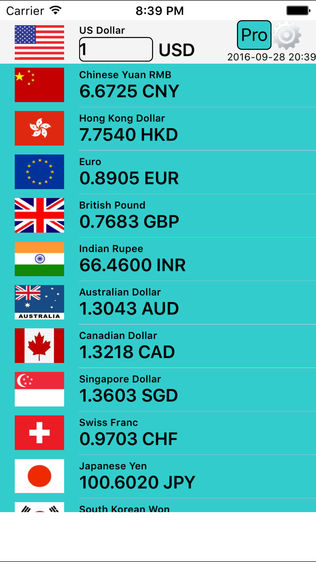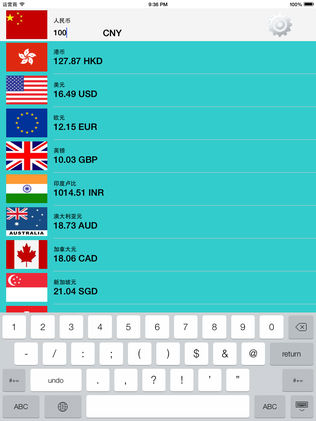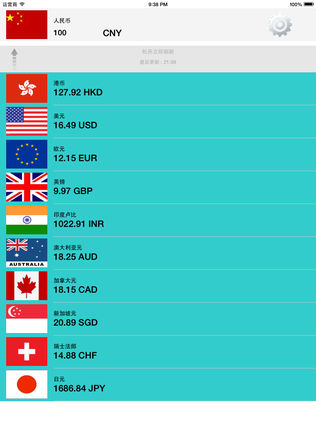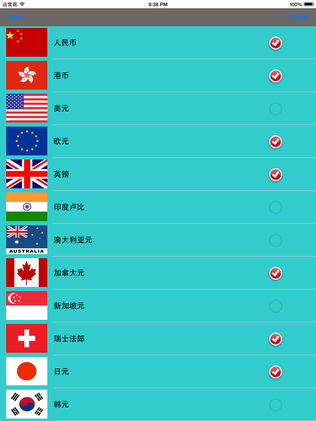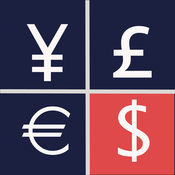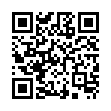【基本介绍】

CNY=人民币;
?HKD=港币;
?USD=美元;
?EUR=欧元;
?GBP=英镑;
?INR=印度卢比;
?AUD=澳大利亚元;?
SGD=新加坡元;
?CHF=瑞士法郎;
?JPY=日元;
?KRW=韩元;
?MOP=澳门元;
?MYR=马来西亚林吉特;?
NZD=新西兰元;
?THB=泰铢;
?HU
【基本介绍】

CNY=人民币;
?HKD=港币;
?USD=美元;
?EUR=欧元;
?GBP=英镑;
?INR=印度卢比;
?AUD=澳大利亚元;?
SGD=新加坡元;
?CHF=瑞士法郎;
?JPY=日元;
?KRW=韩元;
?MOP=澳门元;
?MYR=马来西亚林吉特;?
NZD=新西兰元;
?THB=泰铢;
?HUF=匈牙利福林;
?AED=阿联酋迪拉姆;
?MXN=墨西哥比索;
?ZAR=南非兰特;
BSD=巴哈马元;
?XDR=国际货币基金组织特别提款权;
?RWF=卢旺达法郎;
?AWG=阿鲁巴盾;?
DJF=吉布提法郎;
?BTN=不丹努尔特鲁姆;?
KMF=科摩罗法郎;
?WST=萨摩亚塔拉;?
SPL=塞波加大公国Luigino;
?ERN=厄立特里亚纳克法;?
FKP=福克兰群岛镑;
?SHP=圣赫勒拿镑;
?JEP=泽西岛镑;
?TMT=土库曼斯坦马纳特;
?TVD=图瓦卢元;?
IMP=曼岛镑;
?GGP=根西岛镑;
PLN=波兰兹罗提;
?QAR=卡塔尔里亚尔;
?XAU=金（盎司）;
?OMR=阿曼里亚尔;?
COP=哥伦比亚比索;
?CLP=智利比索;
?TWD=新台币;
?ARS=阿根廷比索;?
CZK=捷克克朗;
?VND=越南盾;?
?JOD=约旦第纳尔;
?BHD=巴林第纳尔;
?XOF=CFA法郎;?
LKR=斯里兰卡卢比;
PHP=菲律宾比索;
?SEK=瑞典克朗;
?IDR=印度尼西亚卢比;
?SAR=沙特里亚尔;
?BRL=巴西雷亚尔;
?TRY=土耳其里拉;
?KES=肯尼亚先令;
?EGP=埃及镑;
?IQD=伊拉克第纳尔;
?NOK=挪威克朗;
?KWD=科威特第纳尔;
?RUB=俄罗斯卢布;?
DKK=丹麦克朗;?
PKR=巴基斯坦卢比;
?ILS=以色列谢克尔;
?XPD=钯（盎司）;
?SVC=萨尔瓦多科朗;?
?UAH=乌克兰格里夫纳;?
NGN=尼日利亚奈拉;
?TND=突尼斯第纳尔;
?UGX=乌干达先令;
?RON=罗马尼亚新列伊;?
BDT=孟加拉国塔卡;
?PEN=秘鲁新索尔;
?GEL=格鲁吉亚拉里;
?XAF=中非金融合作法郎;
?FJD=斐济元;
?VEF=委内瑞拉玻利瓦尔;
?BYR=白俄罗斯卢布;
?HRK=克罗地亚库纳;
?UZS=乌兹别克斯坦索姆;?
BGN=保加利亚列弗;
?DZD=阿尔及利亚第纳尔;
?IRR=伊朗里亚尔;
?DOP=多米尼加比索;?
ISK=冰岛克朗;
?XAG=银（盎司）;
?CRC=哥斯达黎加科朗;
?SYP=叙利亚镑;
?LYD=利比亚第纳尔;
?JMD=牙买加元;
?MUR=毛里塔尼亚卢比;?
GHS=加纳塞地;
?AOA=安哥拉宽扎;
?UYU=乌拉圭比索;
?AFN=阿富汗尼;?
ZMW=赞比亚克瓦查;
?LBP=黎巴嫩镑;
?XPF=CFP法郎;
?LVL=拉脱维亚拉特;
?TTD=特立尼达元;
?LTL=立陶宛立特;
?TZS=坦桑尼亚先令;?
ALL=阿尔巴尼列克;
?XCD=东加勒比元;?
GTQ=危地马拉格查尔;
?NPR=尼泊尔卢比;
?BOB=玻利维亚诺;
?ZWD=津巴布韦元;
?BBD=巴巴多斯元;
?CUC=古巴可兑换比索;
?LAK=老挝基普;
?BND=文莱元;
?BWP=博茨瓦纳普拉;
?HNL=洪都拉斯伦皮拉;
?PYG=巴拉圭瓜拉尼;
??ETB=埃塞俄比亚比尔;
?PGK=巴布亚新几内亚基那;?
SDG=苏丹镑;
?NIO=尼加拉瓜科多巴;?
BMD=百慕大元;
?KZT=哈萨克斯坦坚戈;
?PAB=巴拿马巴波亚;
?BAM=波斯尼亚可兑换马尔卡;
?GYD=圭亚那元;?
YER=也门里亚尔;?
MGA=马达加斯加阿里亚里;
?KYD=开曼元;
?MZN=莫桑比克梅蒂卡尔;
?RSD=塞尔维亚第纳尔;
?SCR=塞舌尔卢比;
?AMD=亚美尼亚德拉姆;
?SBD=所罗门群岛元;?
AZN=阿塞拜疆新马纳特;
?SLL=塞拉利昂利昂;?
TOP=汤加潘加;?
BZD=伯利兹元;
?MWK=马拉维克瓦查;
?GMD=冈比亚达拉西;
?BIF=布隆迪法郎;?
SOS=索马里先令;
?HTG=海地古德;
?GNF=几内亚法郎;
?MVR=马尔代夫拉菲亚;?
MNT=蒙古图格里克;
?CDF=刚果法郎;
?STD=圣多美多布拉;?
TJS=塔吉克斯坦索莫尼;
?KPW=朝鲜元;
?MMK=缅元;?
LSL=莱索托洛蒂;
?LRD=利比亚元;
?KGS=吉尔吉斯斯坦索姆;?
GIP=直布罗陀镑;
?XPT=铂（盎司）;?
MDL=摩尔多瓦列伊;
?CUP=古巴比索;
?KHR=柬埔寨瑞尔;
?MKD=马其顿第纳尔;?
VUV=瓦努阿图瓦图;
?MRO=毛里塔尼亚乌吉亚;
?ANG=荷兰盾;
?SZL=斯威士兰里兰吉尼;
?CVE=佛得角埃斯库多;
?SRD=苏里南元;

【更新日志】
DesignedforiPhoneXS
[展开全部↓]